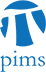## Algebraic Geometry Seminar: Arc spaces and equivariant cohomology

• Date: 11/09/2009
Lecturer(s):
Alan Stapledon (MSRI)
Location:

University of British Columbia

Description:

In the first of a two lecture series (to be completed by Dave Anderson immediately following), we present a new geometric interpretation of equivariant cohomology in which one replaces a smooth, complex $G$-variety $X$ by its associated arc space $J_{\infty} X$, with its induced $G$-action. If $X$ admits an `equivariant affine paving', then we deduce an explicit geometric basis for the equivariant cohomology ring. Moreover, under appropriate hypotheses, we obtain explicit bijections between bases for the equivariant cohomology rings of smooth varieties related by an equivariant, properbirational map. As an initial application, we present a geometric basis for the equivariant cohomology ring of a smooth toric variety.

Schedule:

3:10-4:00pm, WMAX 110.